Courses
Courses for Kids
Free study material
Offline Centres
More

# Thermal Physics - Physics JEE AdvancedLast updated date: 04th Dec 2023
Total views: 20.1k
Views today: 0.20k## Concepts of Thermal Physics for JEE Advanced Physics

The chapter Thermal Physics covers the most important concepts for JEE Advanced and other competitive exams. In the Thermal Physics chapter, students will study the basic heat transfer process and some laws related to the chapter.

The chapter on thermal physics begins with the very basic definition of Newton’s laws of cooling, the First law of thermodynamics and what are different other laws that are helpful in studying the chapter ahead. These concepts will lead us to a very important part of the chapter i.e. Reversible and Irreversible processes, Carnot engine, and efficiency. In this chapter, we will get to study many interesting and most important concepts such as thermal expansion, Specific heat capacity and Mean free path.

The concepts mentioned above will help us in studying the chapter  deeply along with the hands-on practice of many important numerical problems that will help us in acing the exams.

In this article, we will cover the important concepts and topics that will help students to revise and boost their preparations for JEE.

## JEE Advanced Physics Chapters 2024

 JEE Advanced Physics Chapters 1 General Physics 5 Electromagnetic Waves 2 Mechanics 6 Optics 3 Thermal Physics 7 Modern Physics 4 Electricity and Magnestism

### Important Topics of Thermal Physics Chapter

• Heat Transfer

• First Law of Thermodynamics and Heat Capacity

• Second Law of Thermodynamics

• Newton’s Law of Cooling

• Reversible and Irreversible Processes

• Carnot Engine

• Temperature and Thermal Expansion

• Specific Heat Capacity and Mean Free Path

• Thermodynamic Variables

### Thermal Physics Important Concept for JEE Advanced

 Name of the Concept Key Points Heat Transfer This process of heat transfer takes place in three formsConduction( this involves physical contact between two objects such that the heat transfer takes place from warmer body to cooler body)Convection( this takes place in the form of convection currents and it doesn’t require any physical touch between two bodies.)Radiation( this takes place in the form of rays that lie in the electromagnetic spectrum.) Newton’s Law of Cooling This law states that the heat loss from anybody is directly proportional to the difference in temperature in the body and the corresponding surroundings.The formula for the law is given by$\dfrac{dT}{dt} = -k(T-T_{s})$ Zeroth Law of Thermodynamics The law states that two systems in thermal equilibrium with a third system separately are in thermal equilibrium with each other. First Law of Thermodynamics This law states that the energy supplied to the system goes in part to increase the internal energy of the system and rest in work done on the surroundings.The above statement can be formulated as-$\triangle Q$ = $\triangle U$ + $\triangle W$This law is in accordance with the Law of Conservation of Energy. Heat Capacity Heat Capacity denoted by “ S” is defined as the minimum amount of heat required to raise the temperature of a substance from T to T + $\triangle T$This is a mass-dependent property.S = $\dfrac{\triangle Q}{\triangle T}$ Specific Heat Capacity Specific heat capacity denoted by “s” is defined ass = $\dfrac{S}{m}$ or $\dfrac{\triangle Q}{m \triangle T}$This clearly shows that specific heat capacity is independent of mass and is a characteristic value of substance only. Thermodynamic  State Variables These variables are of two types:-Extensive - These are those variables that are dependent on the mass of the system or the amount of quantity present in that system. E.g. Internal Energy, Volume and Mass.Intensive- These quantities are independent of the mass of the system like temperature, Pressure, Density. Cyclic Process This process is defined as if a system returns to its initial state after performing a continuous cycle such that the change in internal energy is zero. Heat Engine Heat engine is a device through which the cyclic process takes place in a system.It consists of a working substance which itself is a system.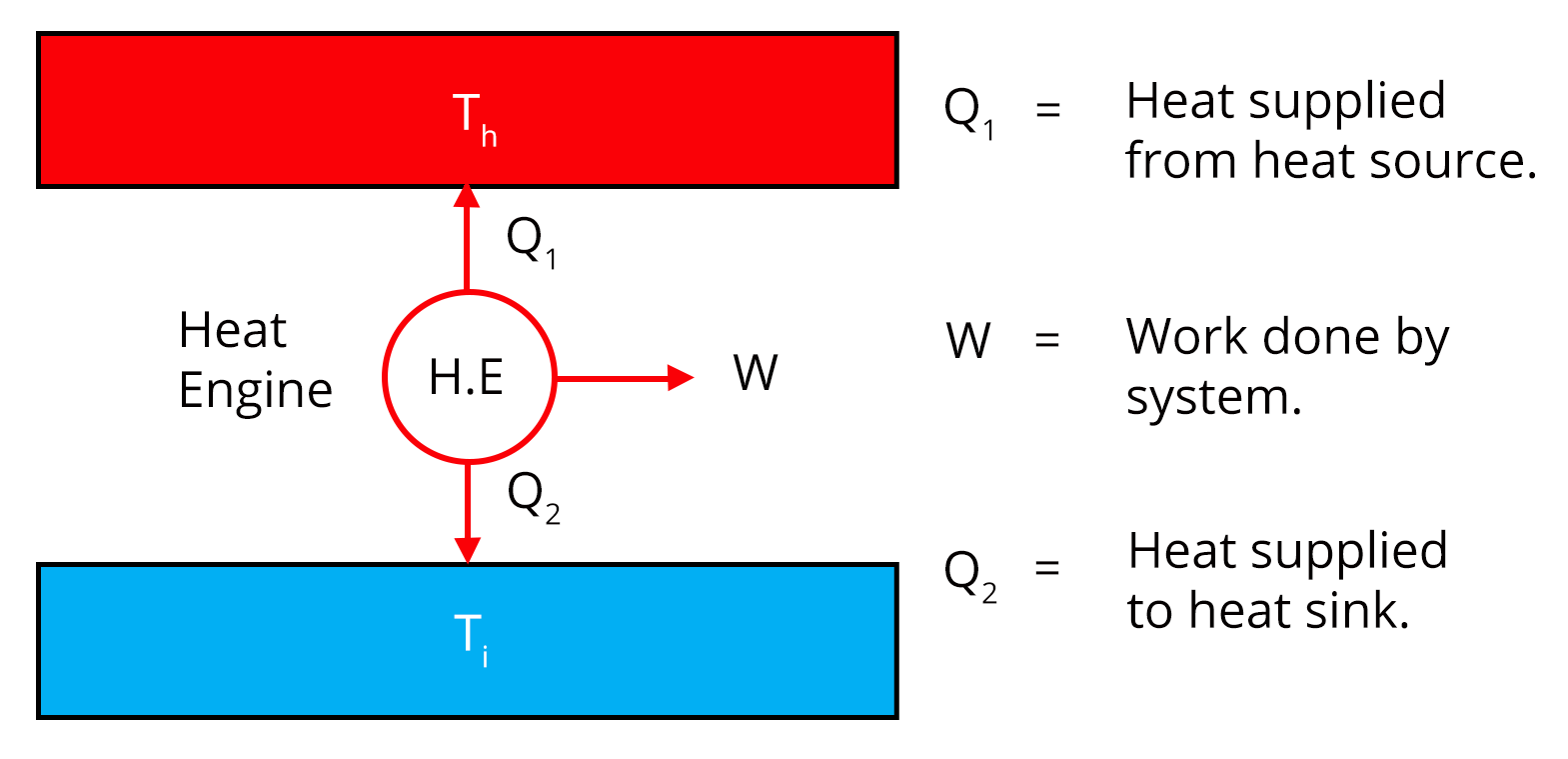If a heat engine has a source i.e. a hot reservoir having heat $Q_{1}$  at temp $T_{1}$ and a sink i.e. cold reservoir which releases heat $Q_{2}$ at temp $T_{2}$  then its efficiency is defined as$\eta = \dfrac{W}{Q_{1}}$Where W is the work done by the system in a cyclic process. Second Law of Thermodynamics The law states that heat can’t be transferred from a body at a lower temperature to a body at a higher temperature without any addition of energy. Reversible and Irreversible processes A thermodynamic process can be said to be reversible if the process returns to its original position from the initial i.e. both system and surroundings return to their original states without any change in the universe.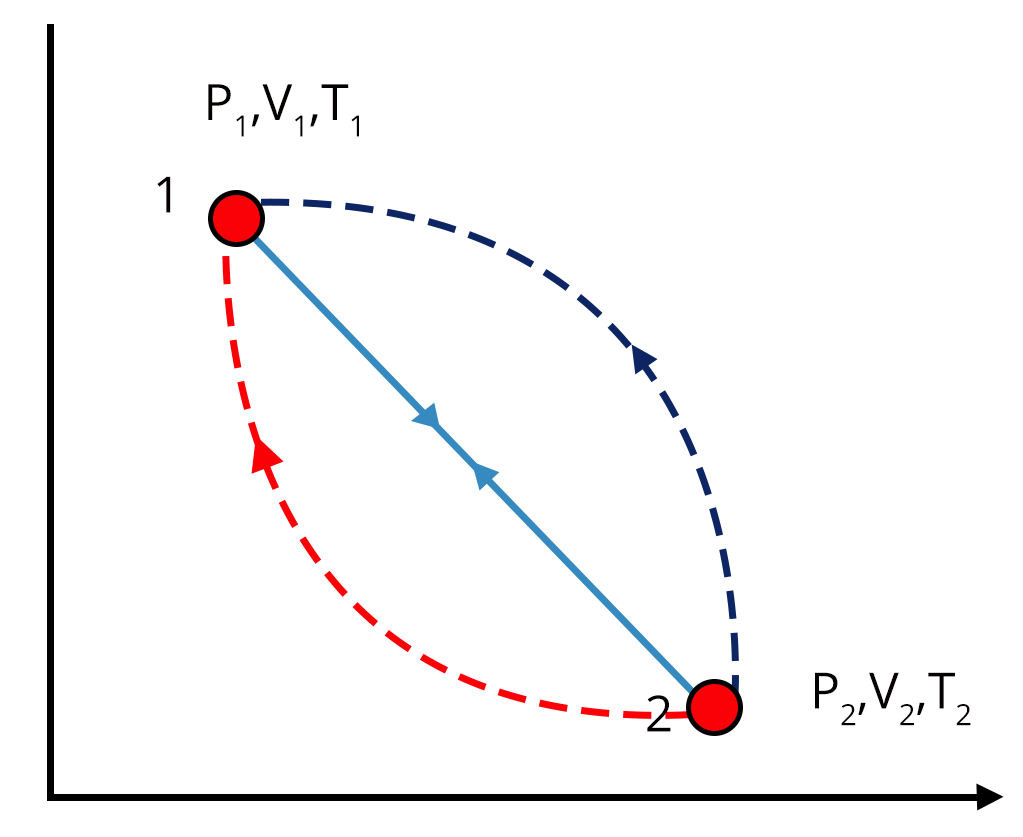Irreversible Processes are those that cannot return to their original conditions with the same path if reversed.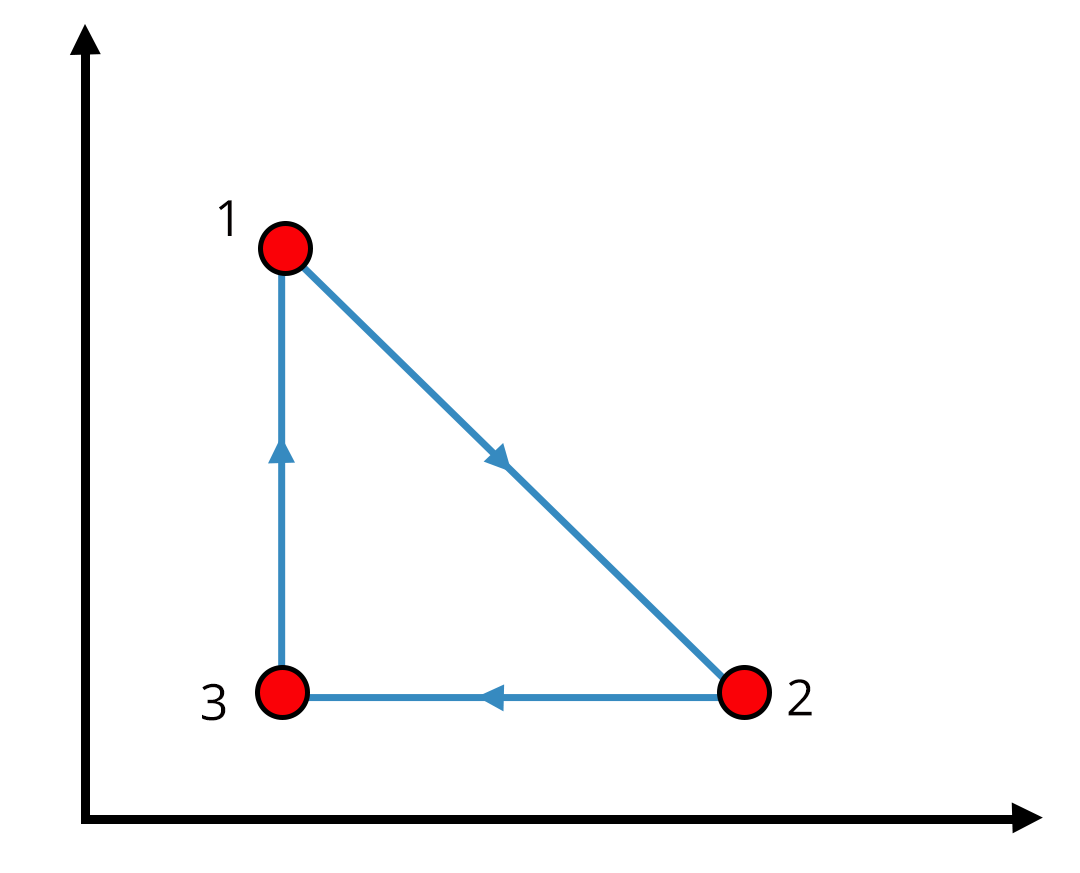Carnot Engine The Carnot engine is the only possible reversible that works between two reservoirs at different temperatures. Efficiency of Carnot engine is given as$\eta = 1-\dfrac{T_{2}}{T_{1}}$Another major formula used in Carnot Cycle which shows the interdependence of system is$\dfrac{T_{1}}{T_{2}}$ = $\dfrac{Q_{1}}{Q_{2}}$ Processes in Carnot Cycle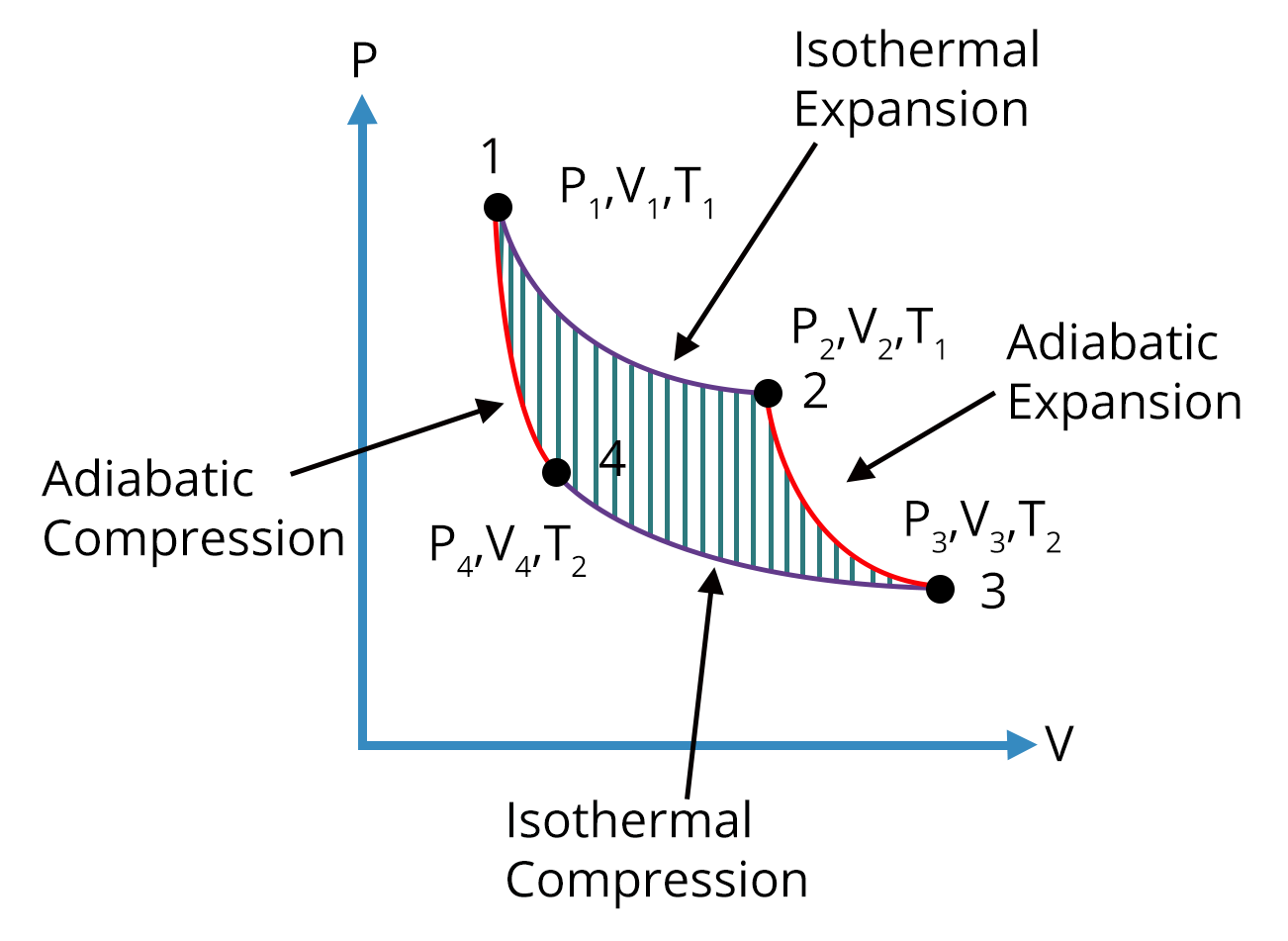Process 1-2 is Isothermal Expansion taking place at a constant temperature.Process 2-3 is Adiabatic Expansion such that no heat is absorbed or lost by the system.Process 3-4 is Isothermal Compression where heat is rejected by the system.Process 4-1 is Adiabatic Compression. Distribution of Molecular Speeds For a given mass of gas the velocities of all molecules are not same even their macroscopic parameters are fixed hence Maxwell introduced various speeds likeMost probable speed-The speed which is attained by large no of molecules having value $v_{p} = 1.41\sqrt{\frac{kT}{m} }$Average speed- Average sum of all the speeds of molecules with value $v_{a} = 1.59\sqrt{\frac{kT}{m} }$Root mean squared - Value of this speed is $v_{rms} = 1.73\sqrt{\frac{kT}{M} }$ Thermal Expansion A phenomenon in which substances expand on heating and contract on cooling with a change in their dimension is called Thermal Expansion. Depending on the shape of the body expansion is of three typesLinear ExpansionArea ExpansionVolume Expansion Thermal Expansion of Solids, Liquids, and Gases Thermal expansion is the phenomenon by which a material expands or contracts in response to changes in temperature. The formula for linear expansion of solids is given by:ΔL = α * L * ΔTWhere:ΔL = Change in lengthα = Coefficient of linear expansionL = Original lengthΔT = Change in temperatureSimilarly, for volume expansion in liquids and gases, the coefficients of volume expansion (β) and cubic expansion (γ) are used. The corresponding formulas are:ΔV = β * V * ΔT (For liquids)ΔV = γ * V * ΔT (For gases) Calorimetry and Latent Heat Calorimetry involves the measurement of heat transfer during chemical reactions or physical processes. The specific heat capacity (C) of a substance is defined as the amount of heat required to raise the temperature of one gram of the substance by one degree Celsius. The formula for heat transfer (Q) is:Q = m * C * ΔTWhere:Q = Heat transferm = MassC = Specific heat capacityΔT = Change in temperatureLatent heat is the heat absorbed or released during a phase change at constant temperature. The formula for latent heat (Q) is:Q = m * LWhere:Q = Latent heatm = MassL = Latent heat of fusion or vaporization Heat Conduction, Convection, and Radiation Heat conduction is the transfer of heat through a solid due to temperature differences. It is governed by Fourier's Law, which states:Q = (k * A * ΔT) / dWhere:Q = Heat transfer ratek = Thermal conductivityA = Cross-sectional areaΔT = Temperature differenced = ThicknessHeat convection involves the transfer of heat through a fluid (liquid or gas) due to the motion of the fluid itself. It is described by Newton's Law of Cooling.Radiation is the transfer of heat through electromagnetic waves, and blackbody radiation laws, including Kirchhoff's law, Wien's displacement law, and Stefan's law, describe the behavior of ideal radiators. Ideal Gas Laws For an ideal gas, the equation of state combines Boyle's Law, Charles's Law, and Avogadro's Law into the ideal gas law:PV = nRTWhere:P = PressureV = Volumen = Number of molesR = Ideal gas constantT = Temperature (in Kelvin) Blackbody Radiation Blackbody radiation laws describe the behavior of ideal radiators. These laws include absorptive and emissive powers, Kirchhoff's law, Wien's displacement law, and Stefan's law. They are crucial for understanding the thermal radiation of objects.

### Different Thermodynamic Processes

 Isochoric Process Isothermal Process Adiabatic Process Isobaric Process This process takes place at const volume. That process in which the temperature of the system is kept constant is called Isothermal process. This process doesn’t allow any exchange of heat i.e. the system is an insulated one with zero amount of heat absorbed or released. That process in which pressure of the system is kept constant is called Isobaric process. For this process the work done in or by the system is zero.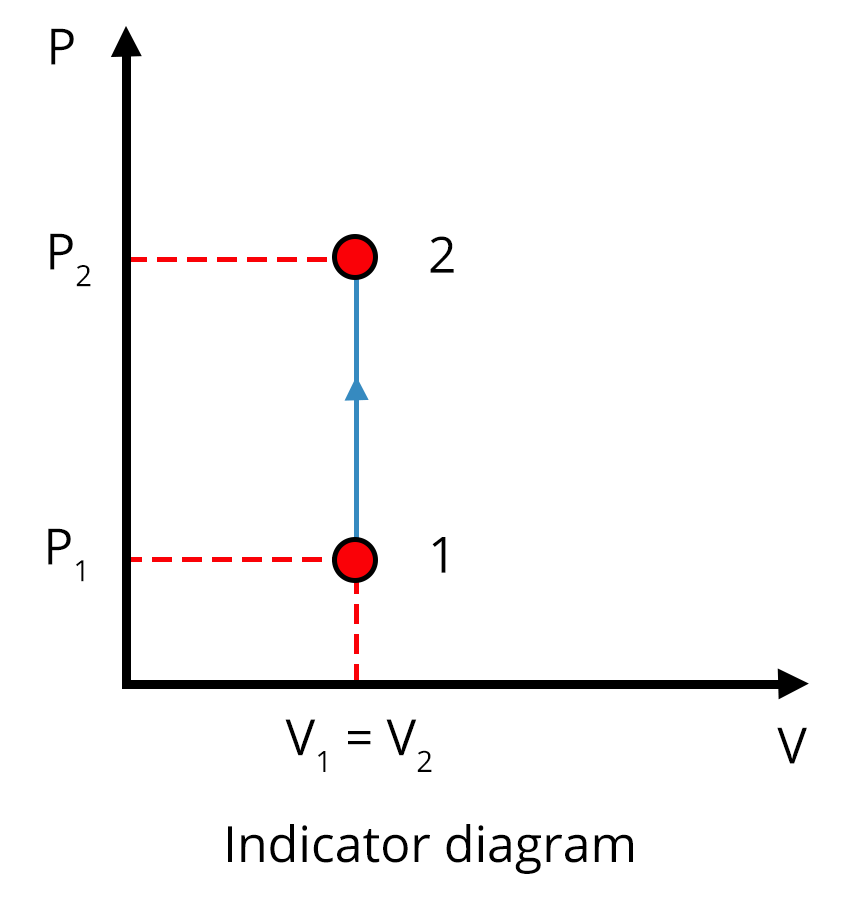Work done for this system is given by$nRT\times 2.303\times\log{\dfrac{V_{2}}{V_{1}}}$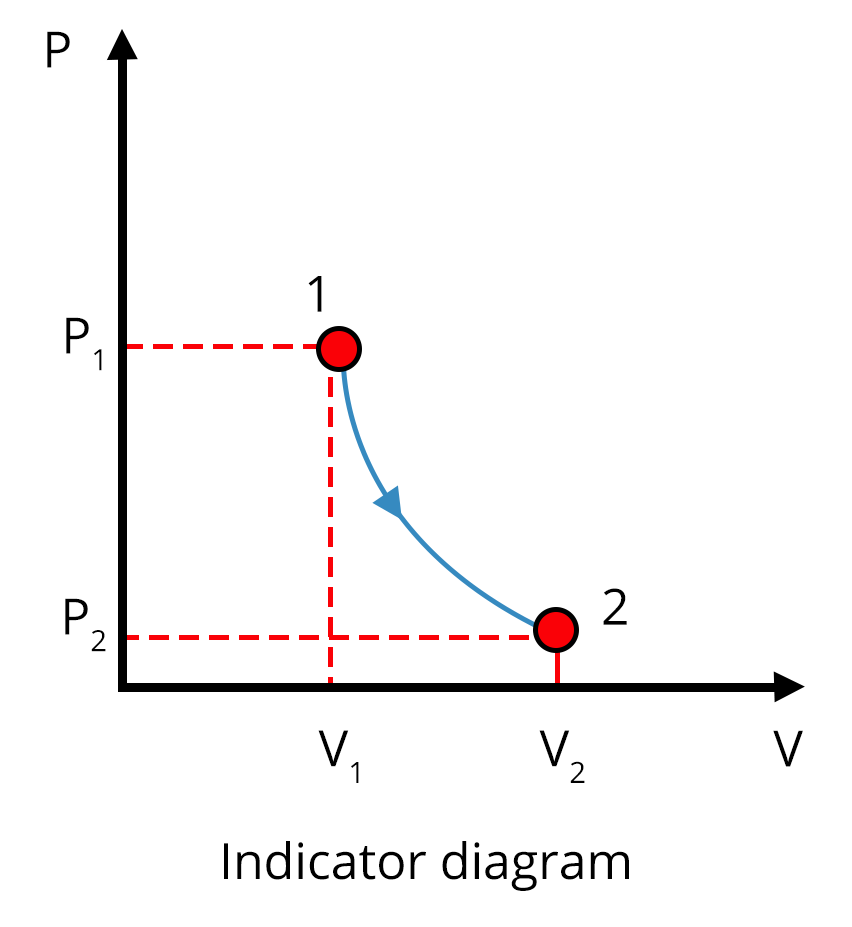Work done for this system is given by$\dfrac{nR(T_{1} - T_{2})}{\gamma -1}$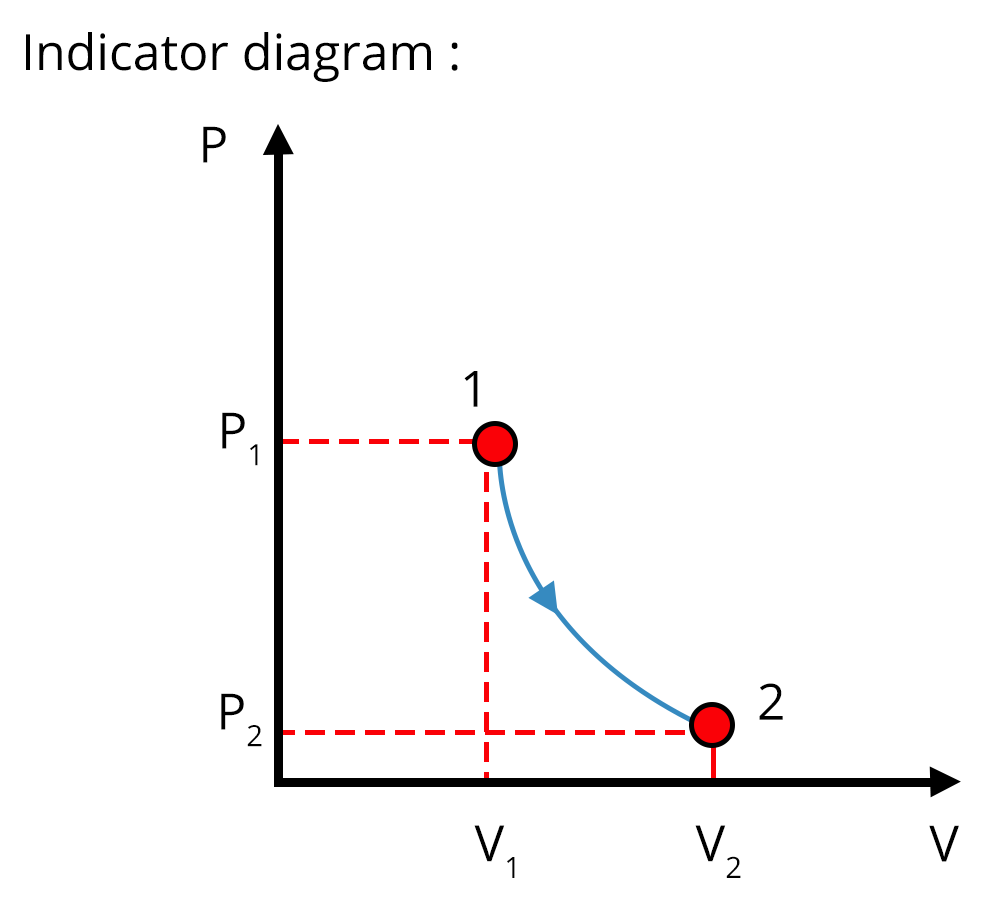Work done by the system for this process is W =nR$(T_{2} - T_{1})$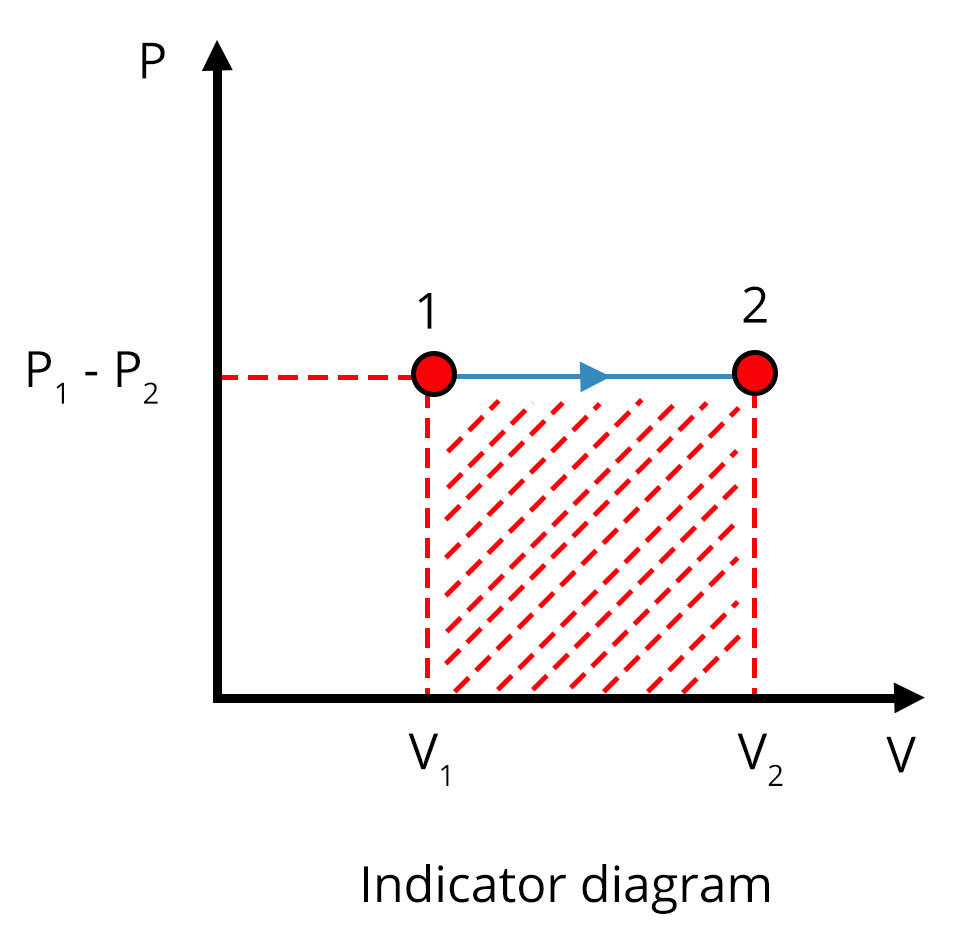### List of Important Formulae of Thermal Physics

 Sl. No Name of the Concept Formulae 1. Newton’s Law of Cooling $\dfrac{dT}{dt} = -k(T-T_{s})$ 2. First Law of Thermodynamics $\triangle Q$ = $\triangle U$ + $\triangle W$ 3. Heat Capacity S = $\dfrac{\triangle Q}{\triangle T}$ 4. Specific Heat Capacity s = $\dfrac{S}{m}$ or $\dfrac{\triangle Q}{m \triangle T}$ 5. Efficiency of heat engine $\eta = \dfrac{W}{Q_{1}}$ 6. Coefficient of Performance $\alpha = \frac{1-\eta}{\eta}$ 7. Work done by Isochoric Process For this process the work done in or by the system is zero. 8. Work done by Isothermal Process $nRT\times 2.303\times\log{\dfrac{V_{2}}{V_{1}}}$ 9. Work done by Isobaric Process W =$nR(T_{2} - T_{1})$ 10. Work done by Adiabatic Process $\dfrac{nR(T_{1} - T_{2})}{\gamma -1}$ 11. Carnot Engine’s Efficiency $\eta = 1-\dfrac{T_{2}}{T_{1}}$ 12. Most probable speed $v_{p} = 1.41\sqrt{\frac{kT}{m} }$ 13. Average speed $v_{a} = 1.59\sqrt{\frac{kT}{m} }$ 14. Root mean squared $v_{rms} = 1.73\sqrt{\frac{kT}{M} }$ 15. Mean free path $\lambda= \dfrac{1}{\sqrt{2}n\pi d^{2}}$ 16. Linear Expansion $\dfrac{\Delta l}{l}= \alpha_{l}\Delta T$

### JEE Advanced Thermal Physics Solved Examples

1. A steam engine delivers 5.4 x 108 J of work per minute and services 3.6 x 109 J of heat per minute from its boiler. What is the efficiency of the engine? How much heat is wasted per minute?

Sol:

Given,

Work done by the boiler per minute (output work) = 5.4 x 108 J

Input work heat absorbed by boiler = 3.6 x 109 J

Hence efficiency = $\eta = \dfrac{\text{output}}{\text{input}}$

= $\dfrac{5.4\times 108} {3.6\times 109}$

=0.15

So the heat wasted per minute is equal to the difference between heat absorbed per minute and output work per minute.

= $\left(3.6 \times {10^9}\right)- \left (5.4 \times {10^8}\right) J$

=$3.06 \times {10^9} J$

Therefore, the efficiency of the engine is 0.15 and heat is wasted per minute.

Key Point: The relationship between the output work and input work is given by relation $\eta = \dfrac{\text{output}}{\text{input}}$

2. A cylinder with a movable piston contains 3 moles of hydrogen at standard temperature and pressure. The walls of the cylinder are made of a heat insulator, and the piston is insulated by having a pile of sand on it. By what factor does the pressure of the gas increase if the gas is compressed to half its original volume?

Sol:

Given,

The cylinder is undergoing adiabatic compression hence for a diatomic gas(hydrogen)  the value of $\gamma$ = 1.4 and as mentioned in the ques final volume = half of the initial one

$V_{2} = \frac{V_{1}}{2}$

$P_{2}$ = 1atm

$P_{1}V_1^\gamma$ = $P_{2}V_2^\gamma$

$V_1^\gamma$ = $P_{2}V_2^\gamma$

$V_1^\gamma$ = $P_{2}(\frac{V_{1}}{2})^{\gamma}$

Hence $P_{2}= 2^{1.4}$

=2.64atm

Therefore, 2.64 atm pressure of the gas increase if the gas is compressed to half its original volume.

Key point: In the case of adiabatic processes, the system is always an insulated one so that no heat can be absorbed or released by the system.

### Previous Year Questions of Thermal Physics

1. Under isothermal conditions, a gas at 300 K expands from 0.1 L to 0.25 L against a constant external pressure of 2 bar . The work done by the gas is( Given that 1L bar=100J)(NEET 2019)

1. 5 kJ

2. 25 J

3. 30 J

4. -30 J

Sol:

Given,

Pressure of the gas = 2bar

Change in volume = $\triangle V$ = 0.25-0.1 = 0.15 L

Since the process is isothermal conditions hence temperature of gas = 0K

From 1st law of thermodynamics

$\triangle Q$ = $\triangle U$ + $\triangle W$

$\triangle Q$  = 0 which means

$\triangle U$ = - $\triangle W$

So the work done is calculated as $\triangle W$ = - $P\triangle V$

=-2$\times$ 0.15 = -0.3L-bar

And as given in ques 1L-bar = 100 J

Hence net work done in Joule is -30J Which is option (d)

Trick: As the given process is isothermal and by the formula of thermodynamics one can see that work done will be negative. So without calculations just by looking at the options, a student can go for an option with a negative sign.

2. A Carnot freezer takes heat from water at $0^{\circ} C$ inside it and rejects it to the room at a temperature of $27^{\circ}C$. The latent heat of ice is $336\times10^{3}$ J/kg. If 5 kg of water at $0^{\circ}C$ is converted into ice at $0^{\circ}C$ by the freezer, then the energy consumed by the freezer is close to:  (JEE 2016)

1. $1.67\times10^{5}$ J

2. $1.68 \times10^{6}$ J

3. $1.51\times10^{5}$ J

4. $1.71 \times10^{7}$ J

Sol:

Given,

Temperature of sink = $T_{sink}$ = $0^{\circ} C$ = 273K

Temperature of source = $T_{source}$ = $27^{\circ} C$ = 300K

Now the latent energy required by freezer to convert ice to water will be the heat rejected by the freezer i.e. $Q_{sink}$ = mL

= 5 $\times 336 \times 10^{3}$ = $1680 \times 10^{3}$ J

From the formula of carnot engine

$\dfrac{T_{sink}}{T_{source}}$ = $\dfrac{Q_{sink}}{Q_{source}}$

Hence $\dfrac{273}{300}$ =$\dfrac{1680 \times 10^{3}}{Q_{source}}$

Or ${Q_{source}}$= $1846.1 \times 10^{3}$ J

So the energy consumed by the freezer = ${Q_{source}}$ - ${Q_{sink}}$

=$1846.1 \times 10^{3}$- $1680 \times 10^{3}$J

= $1.67\times10^{5}$ J which is option (a)

Trick: Here both the conversion of ice into water which is used as latent heat, formula of Carnot engine play an important role in solving the ques.

### Practice Questions

1. A quantity of air at normal temperature is compressed (a) slowly (b) suddenly to one-third of its volume. Find the rise in temperature, if any in each case $\gamma$ = 1.4 (Ans-(a) zero (b) 150.6$^{\circ}C$)

2. The water of one kg mass at 373 K is converted into steam at the same temperature and at atmospheric pressure. On boiling, 1 cc of water takes a volume of 1671 cc. Calculate the change in internal energy of the system taking the heat of vaporization to be 540 cal/g.(Ans- 499.84k cal)

## JEE Advanced Physics Thermal Physics Study Materials

Here, you'll find a comprehensive collection of study resources for Thermal Physics designed to help you excel in your JEE Advanced preparation. These materials cover various topics, providing you with a range of valuable content to support your studies. Simply click on the links below to access the study materials of Thermal Physics and enhance your preparation for this challenging exam.

 JEE Advanced Thermal Physics Study Materials JEE Advanced Thermal Physics Notes JEE Advanced Thermal Physics Important Questions JEE Advanced Thermal Physics Practice Paper

## JEE Advanced Physics Study and Practice Materials

Explore an array of resources in the JEE Advanced Physics Study and Practice Materials section. Our practice materials offer a wide variety of questions, comprehensive solutions, and a realistic test experience to elevate your preparation for the JEE Advanced exam. These tools are indispensable for self-assessment, boosting confidence, and refining problem-solving abilities, guaranteeing your readiness for the test. Explore the links below to enrich your Physics preparation.

### Conclusion

We conclude that the chapter ‘Thermal Physics’ has many important concepts that will help us in scoring better marks in NEET and JEE. In this article, we covered thermal expansion, Heat engines, Efficiency and all other important concepts from the chapter along with solved and previous year questions. Students can test their knowledge with the help of practice questions.

## FAQs on Thermal Physics - Physics JEE Advanced

1. Is Thermal Physics important for the JEE/NEET exam?

Yes, the thermal physics chapter is one of the important chapters for JEE and NEET exams. It carries around 2-3 questions. Due to the formula related questions from this chapter, it becomes easy to solve and secure marks in the exams.

2. Is the thermal physics chapter tough?

No, the thermal physics chapter is simple and easy to understand. The chapter includes the basic concepts that we got introduced to in our elementary school. If you understand the concepts discussed in this chapter and practice related questions then you can successfully attempt the questions asked in this chapter in both NEET and JEE exams.

3. Can I crack NEET by solving the previous years’ questions?

According to experts, applicants who practice previous year's papers for JEE and NEET have a good probability of passing the exam. Anyway, practice is the key to success. Students must focus on solving methods and study conceptually along with the PYQs.## Notice board

JEE News
JEE Blogs